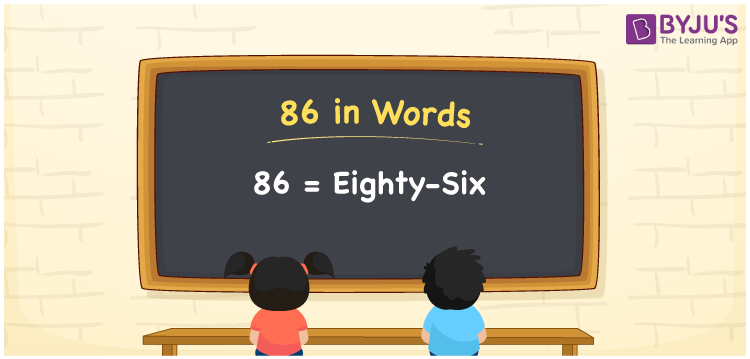# 86 in words

86 in words is written as Eighty Six. 86 represents the count or value. The article on Counting Numbers can give you an idea about count or counting. The number 86 has many factors. It is used at multiple places and instances. For example, “Neetha has a collection of Eight Six pairs of earrings.” Another example, “To lose weight, I need to follow a diet regime for Eighty Six days”.

 86 in words Eighty Six Eighty Six in Numbers 86

## 86 in English Words## How to Write 86 in Words?

We can convert 86 to words using a place value chart. The number 86 has 2 digits. Let us check how 86 would look like in a chart that shows the place value up to 2 digits.

 Tens Ones 8 6

Thus, we can write the expanded form as:

8 × Ten + 6 × One

= 8 × 10 + 6 × 1

= 86

= Eighty Six.

86 is the natural number that is succeeded by 85 and preceded by 87.

86 in words – Eighty Six.

Is 86 an odd number? – No.

Is 86 an even number? – Yes.

Is 86 a perfect square number? – No.

Is 86 a perfect cube number? – No.

Is 86 a prime number? – No.

Is 86 a composite number? – Yes.

## Solved Example

1. Write the number 86 in expanded form

Solution: 8 x 10 + 6 x 1

We can write 86 = 80 + 6

= 8 x 10 + 6 x 1

## Frequently Asked Questions on 86 in words

Q1

### How to write 86 in words?

86 in words is written as Eighty Six.
Q2

### State if True or False. 86 is an even number and hence it is divisible by 2?

True. As 86 is an even number, it is divisible by 2. It is also divisible by 43.
Q3

### Can we express 86 in multiple ways?

Yes. 86 can be expressed as 1 × 86 or 2 × 43